#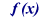Evaluate Expression Module

Used to direct the script to a different module based on the value of Result Variable, Boolean expression or an Arithmetic expression specified. This module is used when the call is to go to different parts of script depending on:

• Caller ID
• Time and Date of the call
• Information entered by caller
• Information retrieved using DB Query / Web Service / Run Program / Run Script modules
• Any VBScript expression (see here for complete list of functions)

The result of the evaluated expression will be assigned to the \$RV[module title] Result Variable.

Optionally the result can also be assigned to a user specified Result Variable. This is useful when implementing counters or other more advanced call flow management.

The name of the user specified Result Variable can be anything. The user specified variable can be accessed in other parts of the script using \$RV[user specified variable] format.

Selecting "Make Global RV" will make the created variable a "Global Result Variable". Global RVs need only be used if many different lines want to be able to read/set a central globally available variable which is retained by VoiceGuide as long as the software is running and does not get reset when any new calls arrive on any lines. (This option is used only in rare circumstances).

Selecting the "Store results in log file" option will ensure the results will be stored along with other call details. If the option is not selected the Result Variables will still be accessible within other parts of the script, but they will not be saved in the call log.

##### Paths Chosen:

If a Result Variable or an Arithmetic expression is specified:

• if any of the paths match the result exactly then that path will be taken.
• If none of the Paths match the value exactly, but the result is non-empty then the "True" path will be taken.
• If the result is empty, then the "False" path will be taken.
• If the result is a '0', then the "False" path will be taken.

If a Boolean expression is specified:

• A "True" or a "False" path will be taken depending on what was the result of the expression.

##### Example 1:

The Evaluate Expression can be used to switch to different parts of the script based on Caller ID. To switch based on the caller's phone number use \$RV_CIDNUMBER as the Result Variable to be evaluated :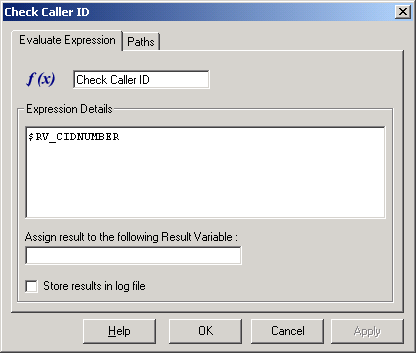And when specifying the paths, use the telephone number in the { } section.

eg: If \$RV_CIDNUMBER was used, and you want to handle calls from number 5625551234 in a special way, the path to send all the calls from this number to a particular module would be:

on {5625551234} goto [List Administrator Options]

you could also use:

on {} goto [NoCallerId]

or

on {False} goto [NoCallerId]

both of which will match if CallerID number is blank.

on {True} goto [CallerIdWasSent]

will be taken if CallerID was provided but no specified path explicitly matched the number: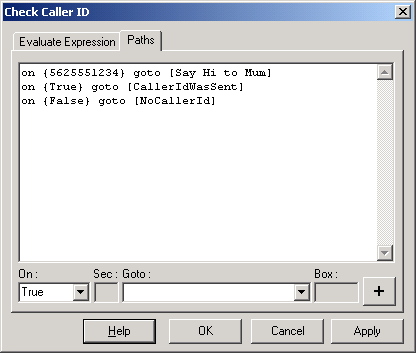##### Example 2:

The Evaluate Expression module is used to check the PIN entered in previous Get Number module titled "Get PIN".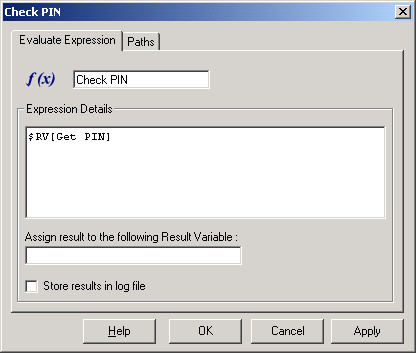And the paths in this case are defined as: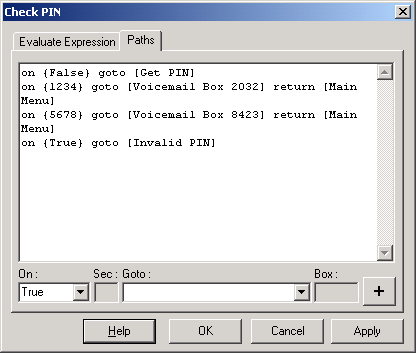What do the paths do:

 on {False} goto [Get PIN] If for some reason no PIN was entered, and \$RV[Get PIN] holds no numbers, then goto the Get PIN module on {1234} goto [Voicemail Box 2032] return [Main Menu] If the entered PIN was 1234 then go to the Voicemail box number 2032, and then when the caller has finished leaving the message return the caller to the Main Menu module on {5678} goto [Voicemail Box 8423] return [Main Menu] If the entered PIN was 5678 then go to the Voicemail box number 8423, and then when the caller has finished leaving the message return the caller to the Main Menu module on {True} goto [Invalid PIN] A PIN was entered and \$RV[Get PIN] does hold some entered number, but that number does not match exactly any of the paths specified. Go to the Invalid PIN module.

It is a good idea to always specify the TRUE and FALSE paths, since VoiceGuide will hang up the call if it cannot find a valid path to go to the next module.

##### Example 3:

The Evaluate Expression module can also be used to perform calculations.

Eg: To calculate whether the number of widgets ordered is more then the available amount you can use the following expression:

(\$RV[LokupStockAvail_1_1]- \$RV[GetOrderAmount]) >= 0

A True or a False path will be taken depending on what was the result of the expression.

##### Example 4:

The Evaluate Expression module can also be used to compare strings. An example of valid expression to evaluate is:

"\$RV[DB_Retrieve_1_1]"="Available"

A True or a False path will be taken depending on what was the result of the above expression

#### Example 5:

If you need to evaluate a Result Variable that holds a string, and select different paths depending on the string’s value then the string will need to be specified using quotes or “CStr()” function:

"\$RV[DB_Retrieve_1_1]"

or:

Cstr(\$RV[DB_Retrieve_1_1])

you will need to use and use the different possible values of this variable when specifying the On ( ‘Paths’ where the script

##### Example 6:

The “+” or the “&” operator can also be used to concatenate strings together.

eg: The following is a valid expression to use:

["1_" & Cstr(\$RV_DAY) & "_2"]

The result is going to be(on a Saturday):

1_6_2

##### Example 7:

If comparing dates the # character should be used around the date expressions.

eg: The following expression determines if current date is between 7th July 2017 and 31 December 2018:

(#7/7/2017# <= #\$RV_MONTH/\$RV_DATE/\$RV_YEAR#) and (#\$RV_MONTH/\$RV_DATE/\$RV_YEAR# <= #31/12/2018#)

##### Example 8:

To add the value of the number entered in module "GetValue1" to the value of the number entered in module "GetValue2" the following expression would be used:

\$RV[GetValue1] + \$RV[GetValue2]

The result will be assigned to the result variable name for the module, or can be assigned to a Result Variable specified in the "Assign result to following Result Variable" field.

##### Example 9:

Evaluate expression can be used as a sophisticated Time-Switch as well. eg. To check if the current day is either New Year's Day or Christmas Day the following expression can be used:

(("\$RV_DD" = "01") and ("\$RV_MM" = "01")) or (("\$RV_DD" = "25") and ("\$RV_MM" = "12"))

##### Example 10:

Evaluate Expression module can be used to implement a counter to check the number of times the caller goes though certain parts of the call flow. This example demonstrates how a counter can be implemented.

A user defined Result Variable is created and its value is set to zero. Note that as the Result Variable was assigned a value of 0, the 'False' path will be taken from this module.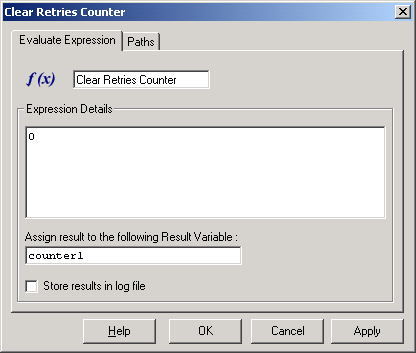The counter in incremented by retrieving the current value of the user defined Result Variable, adding 1 to this current value, and then saving the result back into the user defined Result Variable.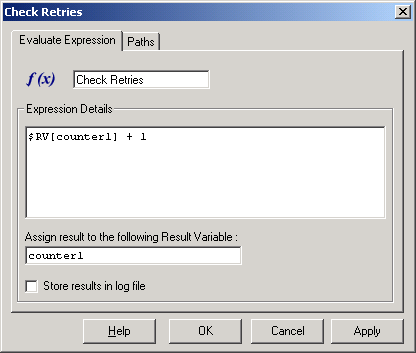The value saved by this Evaluate Expression module is then used in the Paths section to determine the next module the script should branch to.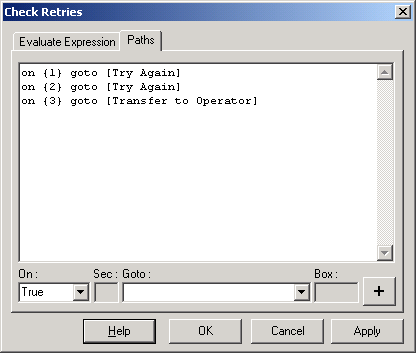##### List of functions which can be used:

Any VBScript type expression can be evaluated using the Evaluate Expression module. The full list of functions/operators/constants/keywords etc which can be used can be found at:

http://www.w3schools.com/asp/asp_ref_vbscript_functions.asp

Functions which can be used in the Evaluate Expression module:

Date/Time Functions
CDate Converts a valid date and time expression to the variant of subtype Date
Date Returns the current system date
DateAdd Returns a date to which a specified time interval has been added
DateDiff Returns the number of intervals between two dates
DatePart Returns the specified part of a given date
DateSerial Returns the date for a specified year, month, and day
DateValue Returns a date
Day Returns a number that represents the day of the month (between 1 and 31, inclusive)
FormatDateTime Returns an expression formatted as a date or time
Hour Returns a number that represents the hour of the day (between 0 and 23, inclusive)
IsDate Returns a Boolean value that indicates if the evaluated expression can be converted to a date
Minute Returns a number that represents the minute of the hour (between 0 and 59, inclusive)
Month Returns a number that represents the month of the year (between 1 and 12, inclusive)
MonthName Returns the name of a specified month
Now Returns the current system date and time
Second Returns a number that represents the second of the minute (between 0 and 59, inclusive)
Time Returns the current system time
Timer Returns the number of seconds since 12:00 AM
TimeSerial Returns the time for a specific hour, minute, and second
TimeValue Returns a time
Weekday Returns a number that represents the day of the week (between 1 and 7, inclusive)
WeekdayName Returns the weekday name of a specified day of the week
Year Returns a number that represents the year

 Asc Converts the first letter in a string to ANSI code CBool Converts an expression to a variant of subtype Boolean CByte Converts an expression to a variant of subtype Byte CCur Converts an expression to a variant of subtype Currency CDate Converts a valid date and time expression to the variant of subtype Date CDbl Converts an expression to a variant of subtype Double Chr Converts the specified ANSI code to a character CInt Converts an expression to a variant of subtype Integer CLng Converts an expression to a variant of subtype Long CSng Converts an expression to a variant of subtype Single CStr Converts an expression to a variant of subtype String Hex Returns the hexadecimal value of a specified number Oct Returns the octal value of a specified number

 FormatCurrency Returns an expression formatted as a currency value FormatDateTime Returns an expression formatted as a date or time FormatNumber Returns an expression formatted as a number FormatPercent Returns an expression formatted as a percentage

 Abs Returns the absolute value of a specified number Atn Returns the arctangent of a specified number Cos Returns the cosine of a specified number (angle) Exp Returns e raised to a power Hex Returns the hexadecimal value of a specified number Int Returns the integer part of a specified number Fix Returns the integer part of a specified number Log Returns the natural logarithm of a specified number Oct Returns the octal value of a specified number Rnd Returns a random number less than 1 but greater or equal to 0 Sgn Returns an integer that indicates the sign of a specified number Sin Returns the sine of a specified number (angle) Sqr Returns the square root of a specified number Tan Returns the tangent of a specified number (angle)

 Array Returns a variant containing an array Filter Returns a zero-based array that contains a subset of a string array based on a filter criteria IsArray Returns a Boolean value that indicates whether a specified variable is an array Join Returns a string that consists of a number of substrings in an array LBound Returns the smallest subscript for the indicated dimension of an array Split Returns a zero-based, one-dimensional array that contains a specified number of substrings UBound Returns the largest subscript for the indicated dimension of an array

 InStr Returns the position of the first occurrence of one string within another. The search begins at the first character of the string InStrRev Returns the position of the first occurrence of one string within another. The search begins at the last character of the string LCase Converts a specified string to lowercase Left Returns a specified number of characters from the left side of a string Len Returns the number of characters in a string LTrim Removes spaces on the left side of a string RTrim Removes spaces on the right side of a string Trim Removes spaces on both the left and the right side of a string Mid Returns a specified number of characters from a string Replace Replaces a specified part of a string with another string a specified number of times Right Returns a specified number of characters from the right side of a string Space Returns a string that consists of a specified number of spaces StrComp Compares two strings and returns a value that represents the result of the comparison String Returns a string that contains a repeating character of a specified length StrReverse Reverses a string UCase Converts a specified string to uppercase

 CreateObject Creates an object of a specified type Eval Evaluates an expression and returns the result IsEmpty Returns a Boolean value that indicates whether a specified variable has been initialized or not IsNull Returns a Boolean value that indicates whether a specified expression contains no valid data (Null) IsNumeric Returns a Boolean value that indicates whether a specified expression can be evaluated as a number IsObject Returns a Boolean value that indicates whether the specified expression is an automation object RGB Returns a number that represents an RGB color value Round Rounds a number ScriptEngine Returns the scripting language in use ScriptEngineBuildVersion Returns the build version number of the scripting engine in use ScriptEngineMajorVersion Returns the major version number of the scripting engine in use ScriptEngineMinorVersion Returns the minor version number of the scripting engine in use TypeName Returns the subtype of a specified variable VarType Returns a value that indicates the subtype of a specified variable

VBScript Keywords

 Empty Used to indicate an uninitialized variable value. A variable value is uninitialized when it is first created and no value is assigned to it, or when a variable value is explicitly set to empty. Example: Dim x   'the variable x is uninitialized! x="ff"   'the variable x is NOT uninitialized anymore x=Empty   'the variable x is uninitialized! Note: This is not the same as Null!! IsEmpty Used to test if a variable is uninitialized. Example: If (IsEmpty(x)) 'is x uninitialized? Nothing Used to indicate an uninitialized object value, or to disassociate an object variable from an object to release system resources. Example: Set myObject=Nothing Is Nothing Used to test if a value is an initialized object. Example: If (myObject Is Nothing) 'is it unset? Note: If you compare a value to Nothing, you will not get the right result! Example: If (myObject = Nothing) 'always false! Null Used to indicate that a variable contains no valid data. One way to think of Null is that someone has explicitly set the value to "invalid", unlike Empty where the value is "not set". Note: This is not the same as Empty or Nothing!! Example: x=Null 'x contains no valid data IsNull Used to test if a value contains invalid data. Example: if (IsNull(x)) 'is x invalid? True Used to indicate a Boolean condition that is correct (True has a value of -1) False Used to indicate a Boolean condition that is not correct (False has a value of 0)

Operators which can be used in Evaluate Expression module:

 Addition (+) Sum two numbers. And Perform a logical conjunction on two expressions. Assignment (=) Assign a value to a variable or property. Concatenation (&) Force string concatenation of two expressions. Division (/) Divide two numbers and return a floating point result. Eqv Perform a logical equivalence on two expressions. Exponentiation (^) Raise a number to the power of an exponent. Imp Perform a logical implication on two expressions. Integer Division (\) Divide two numbers and return an integer result. Is Compare to object reference values. Mod Divide two numbers and return the remainder. Muliplication (*) Multiply two numbers. Negation (-) Indicate the negative value of a numeric expression. Not Perform logical negation of an expression. Or Perform logical disjunction on two expressions. Xor Perform a logical exclusion on two expressions. Subtraction (-) Find the difference between two numbers.